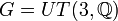# Baer Lie property is not subgroup-closed

This article gives the statement, and possibly proof, of a group property (i.e., Baer Lie group) not satisfying a group metaproperty (i.e., subgroup-closed group property).
View all group metaproperty dissatisfactions | View all group metaproperty satisfactions|Get help on looking up metaproperty (dis)satisfactions for group properties
Get more facts about Baer Lie group|Get more facts about subgroup-closed group property|

## Statement

It is possible to have a Baer Lie group (a 2-powered class two group)$G$ and a subgroup$H$ of$G$ that is not a Baer Lie group.

## Proof

Further information: unitriangular matrix group:UT(3,Q), unitriangular matrix group:UT(3,Z)

Consider the example$G = UT(3,\mathbb{Q})$ and$H = UT(3,\mathbb{Z})$.$G$ is a Baer Lie group.$H$ is a group of class exactly two that is not 2-powered, hence, it is not a Baer Lie group.